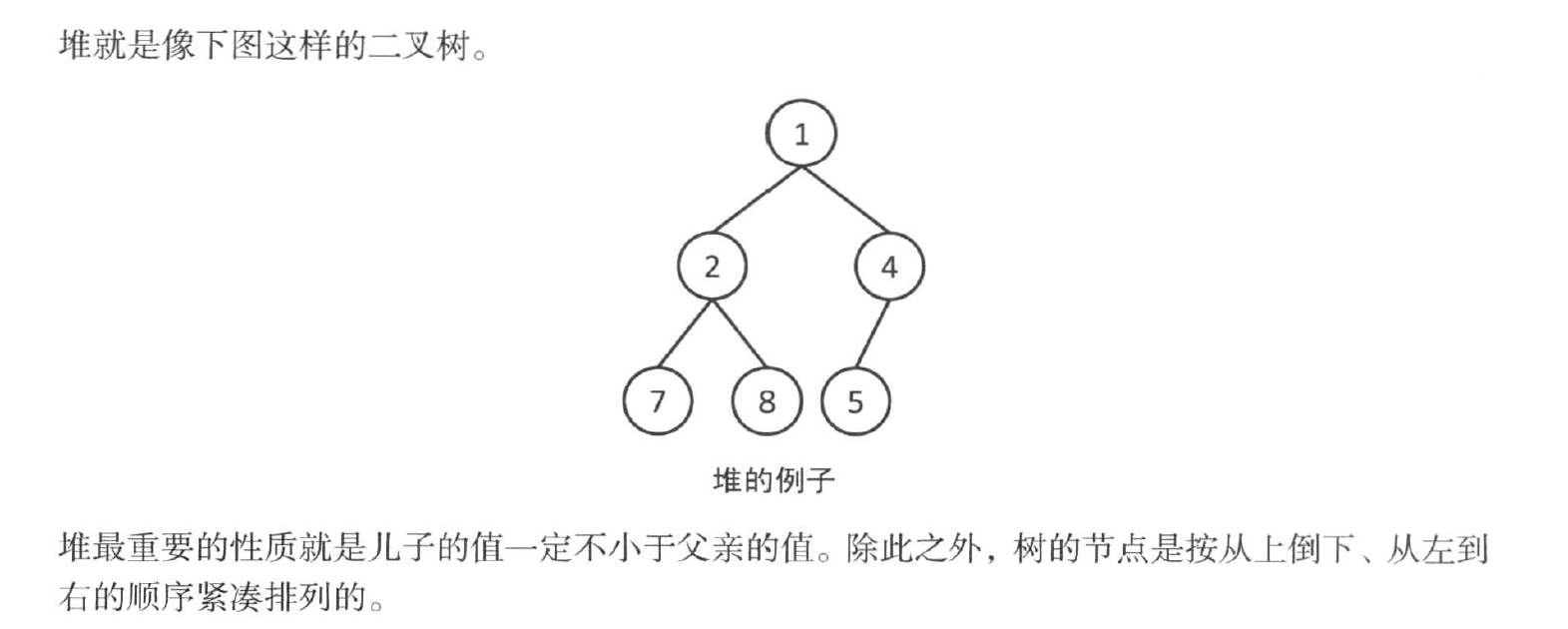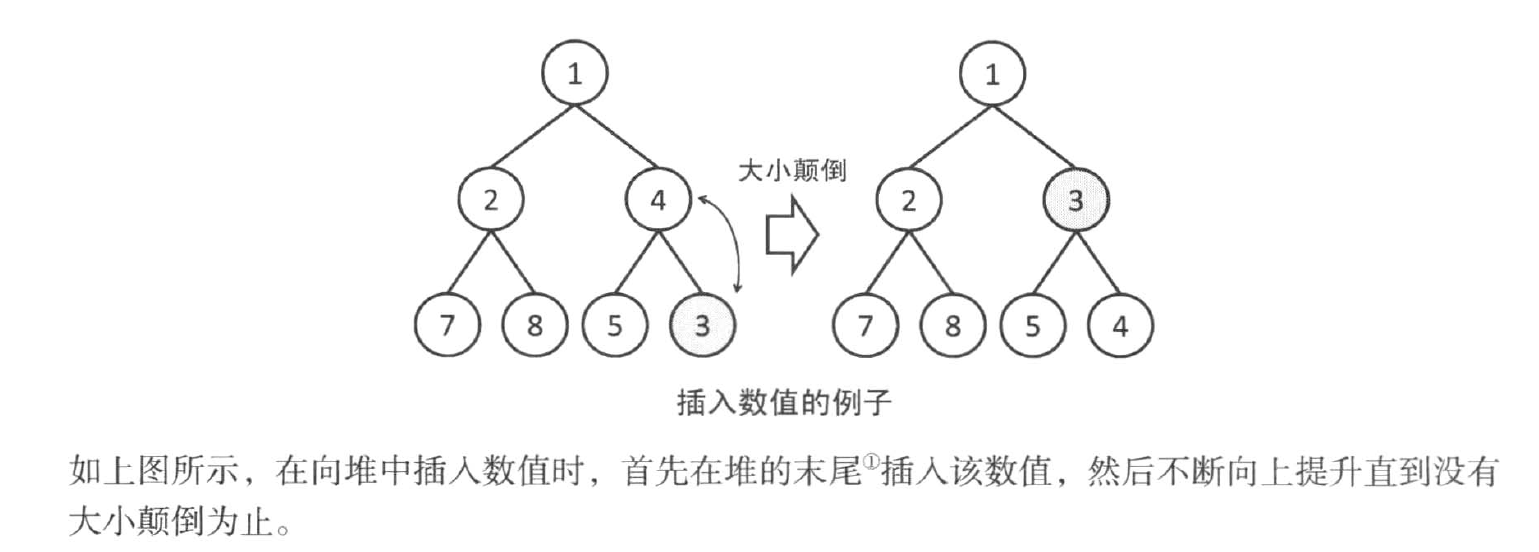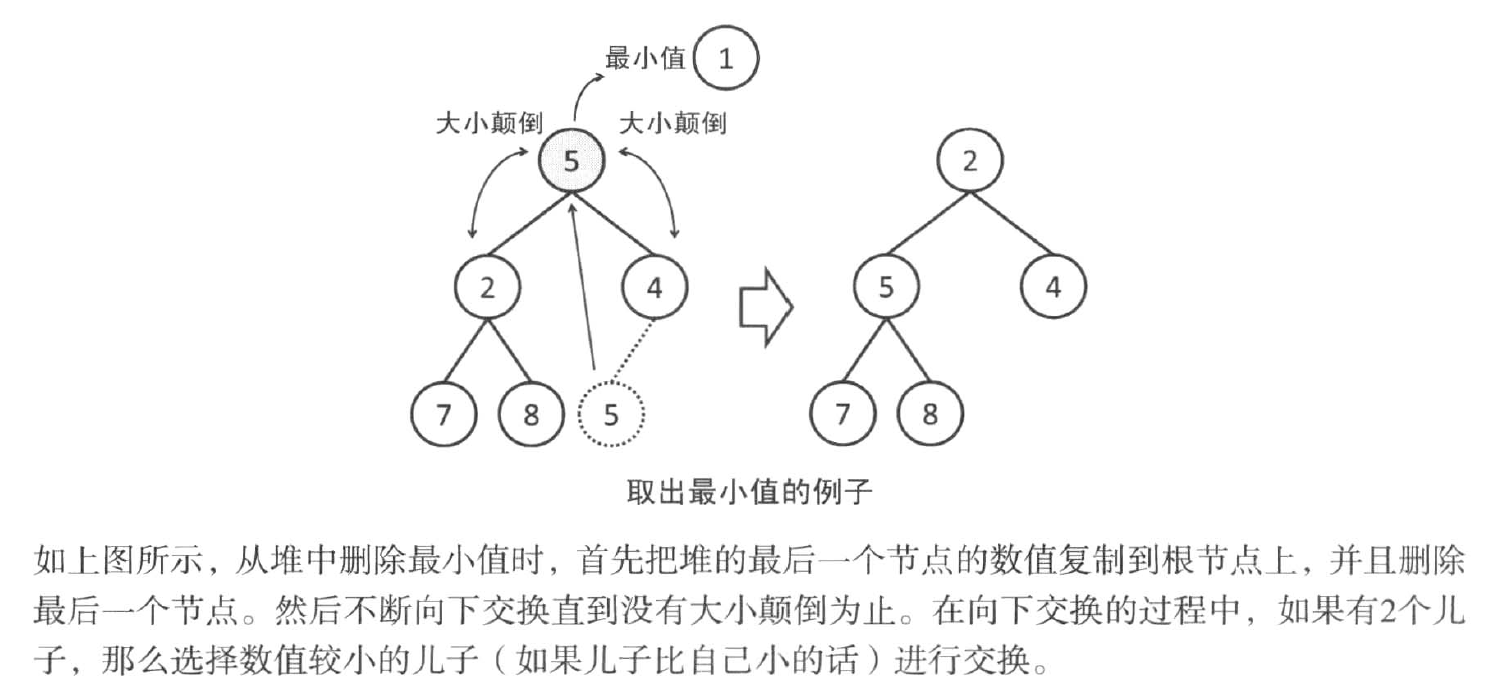# 队列的简单使用

#include<iostream>
#include<queue>
#include<cstdio>
using namespace std;

int main()
{
priority_queue<int> q;//声明一个从到小排序的队列q
q.push(5);//插入值
q.push(8);
q.push(1);
while(!q.empty())//判断至队列为空
{
cout << q.top() << endl;//取队头元素
q.pop();//删除队头元素
}
return 0;
}#define MAX_N 100
int heap[MAX_N],sz = 0;
void push(int x)//heap数组代表代表此时的双亲位置元素，传入的值x为待操作的数
{
//自己节点的编号
int i = sz++;
while(i > 0)
{
//        双亲节点的编号
int p = (i - 1) / 2;
//        如果已经没有大小颠倒则退出
if(heap[p] <= x)
break;
//        把双亲节点的数值放下来，而把自己提上去
heap[i] = heap[p];
i = p;
}
heap[i] = x;
}

int pop()
{
//    ret代表最小值
int ret = heap;
//    要提到根节点的值
int x = heap[sz--];
//    从根开始向下交换
int i = 0;
while(i * 2 + 1 < sz)
{
//        比较儿子的值
int a = i * 2 + 1;//左儿子
int b = i * 2 + 2;//右儿子
if(b < sz && heap[b] < heap[a])
a = b;
//        如果已经没有大小颠倒则退出
if(heap[a] >= x)
break;
//        把儿子的数值提上来
heap[i] = heap[a];
i = a;
}
heap[i] = x;
return ret;
}

posted @ 2019-10-30 19:11  恶魔岛  阅读(...)  评论(...编辑  收藏